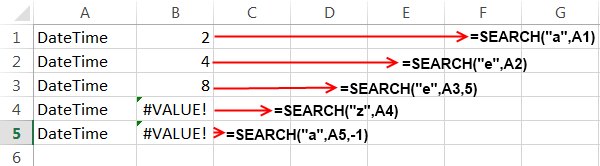# Search Function in Excel – How to Use

As the name suggests Search Function in Excel can help you to locate one string (or character) inside another string. The output of Search function is a number which is equal to the start position of substring inside the input string. Search function when combined with Mid Function can be used as a powerful substring method.

### How Microsoft Excel Defines Search Function:

Microsoft Excel defines Search Function as “It returns the number of the character at which a specific character or text string is first found, reading left to right.”

This means that the Search formula first scans the input string from left to right and then reports the first instance of the character or substring to be searched.

### Syntax of Search function in Excel:

The Syntax of Search function is:

`=SEARCH(substring, text_string, [start_position])`

Here ‘substring’ is the string that you wish to search.

‘text_string’ is the string within which you wish to search.

‘start_position’ is the position in ‘text_string’ from where the search will start. It is an optional argument. If you omit it, then search will start from position 1.

### Some Important Facts about Search Formula:

1. Search function is case-insensitive. This means Search function doesn’t know the difference between ‘D’ and ‘d’.

2. If the ‘substring’ is not found inside ‘text_string’ then Search function will return #VALUE! error.

3. Search Function in excel also supports wildcard operators. We will see an example of this later.

4. If the ‘start_position’ is less than 1 or if it is greater than the length of ‘text_string’, then #VALUE! error is returned.

### Example of Search Formula:

Below is the example of Search Function.In the above example I have used 5 different Search functions and I will be explaining them one by one:

1. In the first function i.e. `=SEARCH("a",A1)`, the formula searches the character “a” inside the string present at A1 position i.e. “DateTime”. The result is 2 because “a” is present at the second position in the string.

2. In the second formula i.e. `=SEARCH("e",A2)`, similar to the first example the character “e” is searched in the string “DateTime”. The function then returns the position of the first instance of “e” which is equal to 4.

3. In the third formula i.e. `=SEARCH("e",A3,5)`, the character “e” is searched in the “DateTime” string but the search starts from position number 5, it encounters “e” at 8th position and hence the result is 8.

4. In the fourth example i.e. `=SEARCH("z",A4)`, the string “z” is searched in the “DateTime” string but as “z” is not present in “DateTime” therefore the search function shows a #VALUE! Error.

5. In the last example i.e. `=SEARCH("a",A5,-1)`, as the start position is less than 1 so the function results into a #VALUE! Error.

### Use of Wildcard Operator in Search:

It is interesting to know that you can also use wildcard operators in the Search Formula. This can be helpful when you are trying to search the strings of a specific pattern.

• Wildcard (?) : denotes any single character.
• Wildcard (*) : denotes any number of characters.In the first example i.e. `=SEARCH("?o?",A1)` matches “som” which is present at the start of the “Some Random text” string and hence the value is 1.

In the next example i.e. `=SEARCH("o*e",A2)` matches “ome” which is starts from the second position in the string “Some Random text” and hence the value is 2.

So, this was all about Search Function available in Excel.About Ankit Kaul

Ankit is the founder of Excel Trick. He is tech Geek who loves to sit in front of his square headed girlfriend (his PC) all day long. :D. Ankit has a strong passion for learning Microsoft Excel. His only aim is to turn you guys into 'Excel Geeks'.